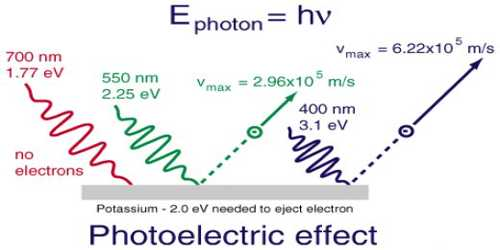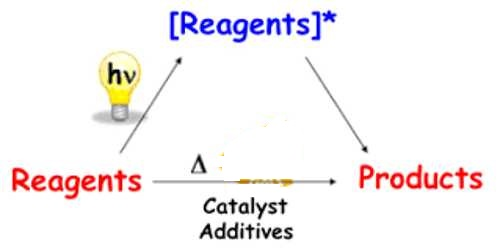Chemistry

# PhotochemistryPhotochemistry

The branch of chemistry which deals with chemical reactions mediated by light is called photochemistry. The term ‘light’ is used to include all kinds of radiation. In practice, however, photochemical studies are confined to the visible and ultraviolet part of the electromagnetic spectrum. The wavelength range of interest is from 180 nm to 700 nm. Infra-red radiation (wavelength > 700 nm) is too weak to bring about a chemical reaction. X-ray (wavelength ≈ 10 nm) produces ionization of molecules and its effect is now-a-days included in the science of radiation chemistry.In dealing with photochemical reactions light may be regarded as small packets of energy or quanta of energy, called the photon. The energy, ɛ of the photon is, given by quantum mechanics as-

ɛ = hv = h (c/λ)

where v is the frequency (v = c/λ) of the radiation, h is the Planck’s constant (h = 6.626 x 10-34 js), c is the velocity of light and λ is the wavelength of radiation. The energy of Avogadro number of light quanta is called an Einstein. The energy corresponding to radiation of a particular wavelength may easily be calculated.

E = Nɛ = Nhv = Nh (c/λ) … … … (1)

If we substitute the values of N (6.022 x 1023 mol-1), h (6.626 x 10-34 Js) and c (3.0 x108 ms-1) in equation (1) we get,

E = [{(6.022 x1023)(6.626×10-34)(3.0×108)} / λ]

E = 0.1197/λ J mol-1

= 11.97 x 10-5 / λ kJ mol-1

Here λ is in meter.